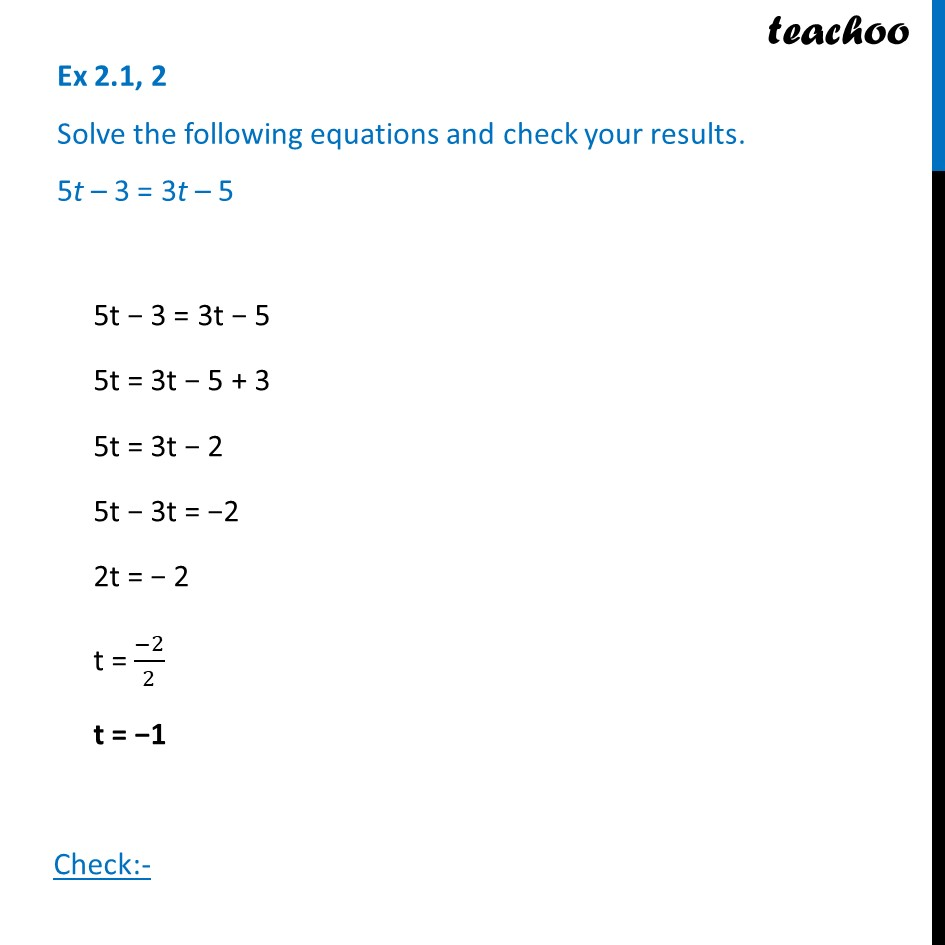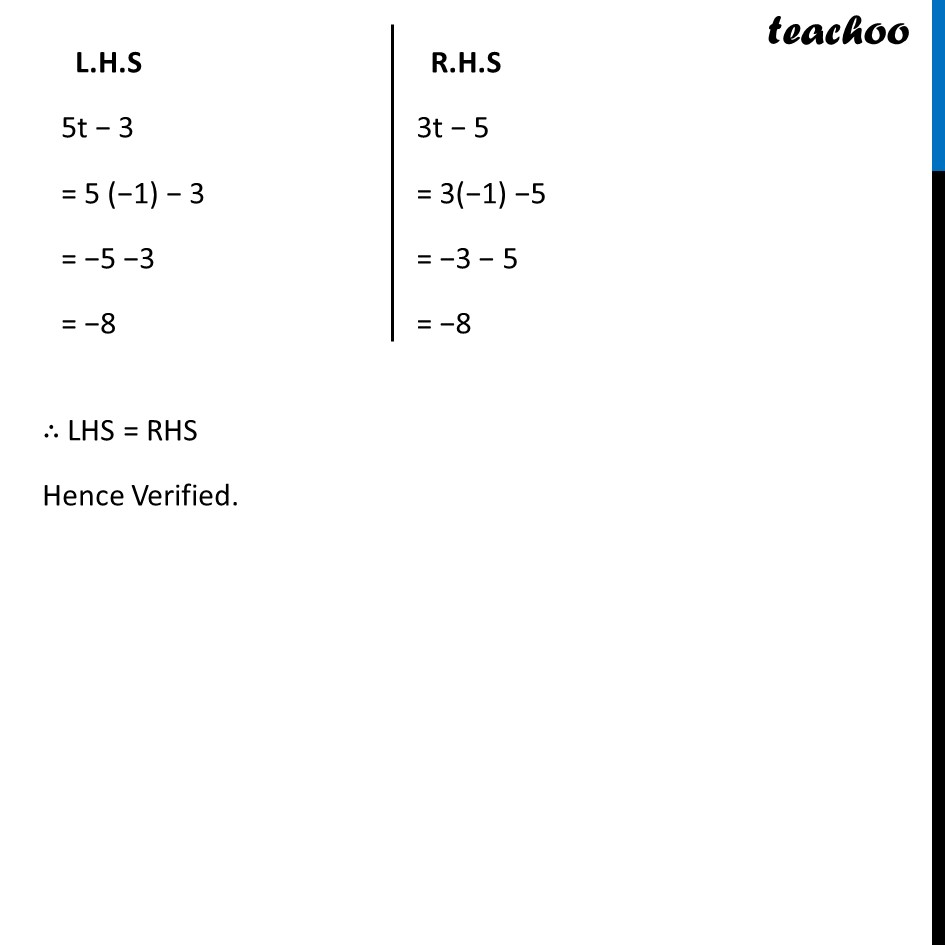Ex 2.1

Chapter 2 Class 8 Linear Equations in One Variable
Serial order wiseLearn in your speed, with individual attention - Teachoo Maths 1-on-1 Class

### Transcript

Ex 2.1, 2 Solve the following equations and check your results. 5t – 3 = 3t – 55t − 3 = 3t − 5 5t = 3t − 5 + 3 5t = 3t − 2 5t − 3t = −2 2t = − 2 t = (−2)/2 t = −1 Check:- L.H.S 5t − 3 = 5 (−1) − 3 = −5 −3 = −8 R.H.S 3t − 5 = 3(−1) −5 = −3 − 5 = −8 ∴ LHS = RHS Hence Verified.